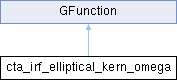GammaLib  1.7.0.dev
cta_irf_elliptical_kern_omega Class Reference

Kernel for ellitpical model azimuth angle IRF integration. More...

#include <GCTAResponse_helpers.hpp>

Inheritance diagram for cta_irf_elliptical_kern_omega:## Public Member Functions

cta_irf_elliptical_kern_omega (const GCTAResponseIrf *rsp, const GModelSpatialElliptical *model, const double &zenith, const double &azimuth, const GEnergy &srcEng, const GTime &srcTime, const GEnergy &obsEng, const double &posangle_obs, const double &omega_pnt, const double &rho, const double &cos_psf, const double &sin_psf, const double &cos_ph, const double &sin_ph)

double eval (const double &omega)
Kernel for elliptical model integration over model's azimuth angle. More...Public Member Functions inherited from GFunction
GFunction (void)
Void constructor. More...

GFunction (const GFunction &function)
Copy constructor. More...

virtual ~GFunction (void)
Destructor. More...

GFunctionoperator= (const GFunction &function)
Assignment operator. More...

## Public Attributes

const GCTAResponseIrfm_rsp
CTA response. More...

const GModelSpatialEllipticalm_model
Spatial model. More...

double m_zenith
Zenith angle. More...

double m_azimuth
Azimuth angle. More...

GEnergy m_srcEng
True photon energy. More...

GTime m_srcTime
True photon time. More...

GEnergy m_obsEng
Measured event energy. More...

double m_posangle_obs
Measured photon position angle from model centre. More...

double m_omega_pnt
Azimuth of pointing in model system. More...

double m_rho
Model zenith angle. More...

double m_cos_psf
Cosine term for PSF offset angle computation. More...

double m_sin_psf
Sine term for PSF offset angle computation. More...

double m_cos_ph
Cosine term for photon offset angle computation. More...

double m_sin_ph
Sine term for photon offset angle computation. More...Protected Member Functions inherited from GFunction
void init_members (void)
Initialise class members. More...

void copy_members (const GFunction &function)
Copy class members. More...

void free_members (void)
Delete class members. More...

## Detailed Description

Kernel for ellitpical model azimuth angle IRF integration.

Kernel for elliptical model azimuth angle integration.

This class implements the computation of

$S_{\rm p}(\rho, \omega | E, t) \, IRF(\rho, \omega)$

where

• $$S_{\rm p}(\rho, \omega | E, t)$$ is the elliptical model,
• $$IRF(\rho, \omega)$$ is the IRF
• $$\rho$$ is the distance from the model centre, and
• $$\omega$$ is the azimuth angle is the position angle with respect to the connecting line between the model centre and the observed photon arrival direction.

This class implements the computation of

$S_{\rm p}(\omega | \rho, E, t) \, PSF(\omega | \rho)$

where $$S_{\rm p}(\omega | \rho, E, t)$$ is the elliptical model, $$PSF(\omega | \rho)$$ is the point spread function, $$\rho$$ is the distance from the model centre, and $$\omega$$ is the position angle with respect to the connecting line between the model centre and the observed photon arrival direction.

Definition at line 538 of file GCTAResponse_helpers.hpp.

## Constructor & Destructor Documentation

 cta_irf_elliptical_kern_omega::cta_irf_elliptical_kern_omega ( const GCTAResponseIrf * rsp, const GModelSpatialElliptical * model, const double & zenith, const double & azimuth, const GEnergy & srcEng, const GTime & srcTime, const GEnergy & obsEng, const double & posangle_obs, const double & omega_pnt, const double & rho, const double & cos_psf, const double & sin_psf, const double & cos_ph, const double & sin_ph )
inline

Definition at line 540 of file GCTAResponse_helpers.hpp.

## Member Function Documentation

 double cta_irf_elliptical_kern_omega::eval ( const double & omega )
virtual

Kernel for elliptical model integration over model's azimuth angle.

Parameters

Computes

$S_{\rm p}(\omega | \rho, E, t) \, IRF(\omega | \rho)$

where $$\omega$$ is the azimuth angle with respect to the model centre, counted counterclockwise from the vector connecting the model centre to the observed photon direction, and $$\rho$$ is the radial distance from the model centre.

From the coordinates $$(\rho,\omega)$$ in the model system, the method computes the angle between the true ( $$\vec{p}$$) and observed ( $$\vec{p'}$$) photon arrival direction using

$\delta = \arccos(\cos \rho \cos \zeta + \sin \rho \sin \zeta \cos \omega)$

where $$\zeta$$ is the angular distance between the observed photon arrival direction $$\vec{p'}$$ and the model centre $$\vec{m}$$. This angle $$\delta$$ is used to compute the $$PSF(\delta)$$ value.

The method computes also the angle $$\theta$$ between the observed photon arrival direction $$\vec{p'}$$ and the camera pointing $$\vec{d}$$ using

$\theta = \arccos(\cos \rho \cos \lambda + \sin \rho \sin \lambda \cos \omega_0 - \omega)$

where $$\lambda$$ is the angular distance between the model centre $$\vec{m}$$ and the camera pointing direction $$\vec{d}$$. The angle $$\theta$$ is used in the computation of the IRF (no azimuthal dependence is so far implemented for the IRF computation).

Implements GFunction.

Definition at line 906 of file GCTAResponse_helpers.cpp.

## Member Data Documentation

 double cta_irf_elliptical_kern_omega::m_azimuth

Azimuth angle.

Definition at line 573 of file GCTAResponse_helpers.hpp.

Referenced by eval().

 double cta_irf_elliptical_kern_omega::m_cos_ph

Cosine term for photon offset angle computation.

Definition at line 582 of file GCTAResponse_helpers.hpp.

Referenced by eval().

 double cta_irf_elliptical_kern_omega::m_cos_psf

Cosine term for PSF offset angle computation.

Definition at line 580 of file GCTAResponse_helpers.hpp.

Referenced by eval().

 const GModelSpatialElliptical* cta_irf_elliptical_kern_omega::m_model

Spatial model.

Definition at line 571 of file GCTAResponse_helpers.hpp.

Referenced by eval().

 GEnergy cta_irf_elliptical_kern_omega::m_obsEng

Measured event energy.

Definition at line 576 of file GCTAResponse_helpers.hpp.

Referenced by eval().

 double cta_irf_elliptical_kern_omega::m_omega_pnt

Azimuth of pointing in model system.

Definition at line 578 of file GCTAResponse_helpers.hpp.

Referenced by eval().

 double cta_irf_elliptical_kern_omega::m_posangle_obs

Measured photon position angle from model centre.

Definition at line 577 of file GCTAResponse_helpers.hpp.

Referenced by eval().

 double cta_irf_elliptical_kern_omega::m_rho

Model zenith angle.

Definition at line 579 of file GCTAResponse_helpers.hpp.

Referenced by eval().

 const GCTAResponseIrf* cta_irf_elliptical_kern_omega::m_rsp

CTA response.

Definition at line 570 of file GCTAResponse_helpers.hpp.

Referenced by eval().

 double cta_irf_elliptical_kern_omega::m_sin_ph

Sine term for photon offset angle computation.

Definition at line 583 of file GCTAResponse_helpers.hpp.

Referenced by eval().

 double cta_irf_elliptical_kern_omega::m_sin_psf

Sine term for PSF offset angle computation.

Definition at line 581 of file GCTAResponse_helpers.hpp.

Referenced by eval().

 GEnergy cta_irf_elliptical_kern_omega::m_srcEng

True photon energy.

Definition at line 574 of file GCTAResponse_helpers.hpp.

Referenced by eval().

 GTime cta_irf_elliptical_kern_omega::m_srcTime

True photon time.

Definition at line 575 of file GCTAResponse_helpers.hpp.

Referenced by eval().

 double cta_irf_elliptical_kern_omega::m_zenith

Zenith angle.

Definition at line 572 of file GCTAResponse_helpers.hpp.

Referenced by eval().

The documentation for this class was generated from the following files: